Function Repository Resource:

# CausalGraphEntanglementEntropyNaive

Compute the entanglement entropy of a certain subgraph of a causal graph (or subset of a causal set) using the naive/fragile approach

Contributed by: Jonathan Gorard and Julia Dannemann-Freitag
 ResourceFunction["CausalGraphEntanglementEntropyNaive"][g,sg,d] computes the entanglement entropy of a specified subgraph sg of a given causal graph g, where g is assumed to be of integer dimension d, using the naive/fragile approach. ResourceFunction["CausalGraphEntanglementEntropyNaive"][g,sg,d,"prop"] gives the property "prop" for the specified naive causal graph entanglement entropy computation.

## Details

Spacetime entanglement entropies are conventionally defined locally on a given spacelike hypersurface; this definition was subsequently extended by Sorkin (2012) to yield a global definition that can be applied to the case of discrete causal graphs (or causal sets). ResourceFunction["CausalGraphEntanglementEntropyNaive"] uses the approach adopted by Sorkin and Yazdi (2018), in which a scalar field is (implicitly) defined over the causal graph/causal set, from which a discrete analog of the Pauli-Jordan and (Sorkin-Johnston) Wightman functions from algebraic quantum field theory can be constructed, whence a discrete spacetime entanglement entropy may subsequently be extracted using their eigenspectra.
ResourceFunction["CausalGraphEntanglementEntropyNaive"] uses the "naive" approach to computing discrete entanglement entropies, in which the (Sorkin-Johnston) Wightman function is inverted and the eigenvalues of the resulting matrix are calculated directly. This is considerably faster than the generalized approach (in which vectors are explicitly constructed that are known to lie strictly within the image of the Pauli-Jordan function, thus solving a more generalized case of an eigenvalue problem), but also much more fragile, since it assumes invertibility of the Wightman function, which will not be the case in general.
When the (Sorkin-Johnston) Wightman function is not invertible, ResourceFunction["CausalGraphEntanglementEntropyNaive"] will return Indeterminate.
In ResourceFunction["CausalGraphEntanglementEntropyNaive"][g,sg,], g is expected to be a directed acyclic graph (representing e.g. a Wolfram model causal graph, or the Hasse diagram of a causal set), and sg is expected to be a proper subgraph of g.
The value of the dimension parameter d determines which form of the causal set Green's function to use, and hence the form of the Pauli-Jordan and Wightman functions subsequently computed. Currently, the Green's functions for 1+1-dimensional, 2+1-dimensional and 3+1-dimensional discrete spacetimes are supported by ResourceFunction["CausalGraphEntanglementEntropyNaive"].
If the dimension parameter d is not specified, it is assumed to be 2 by default.
The techniques used by ResourceFunction["CausalGraphEntanglementEntropyNaive"] are numerically sensitive, and highly dependent upon the underlying linear algebraic algorithms used for constructing and solving the required eigenvalue problem, so some results may differ (both qualitatively and quantitatively) between different versions of the Wolfram Language.
In ResourceFunction["CausalGraphEntanglementEntropyNaive"][,"prop"], the following properties can be requested:
 "EntanglementEntropy" the entanglement entropy of the specified subgraph (default) "CausalGraph" a rendering of the (transitive reduction of the) specified causal graph "FullCausalGraph" a rendering of the (transitive closure of the) specified causal graph "CausalSubgraph" a rendering of the (transitive reduction of the) specified subgraph of the overall causal graph "FullCausalSubgraph" a rendering of the (transitive closure of the) specified subgraph of the overall causal graph "CausalGraphHighlighted" a rendering of the (transitive reduction of the) specified causal graph with the interior subgraph highlighted "FullCausalGraphHighlighted" a rendering of the (transitive closure of the) specified causal graph with the interior subgraph highlighted

## Examples

### Basic Examples (4)

Construct a causal graph by randomly sprinkling 50 points within a diamond-shaped region of 1+1-dimensional Minkowski space:

 In:=Out=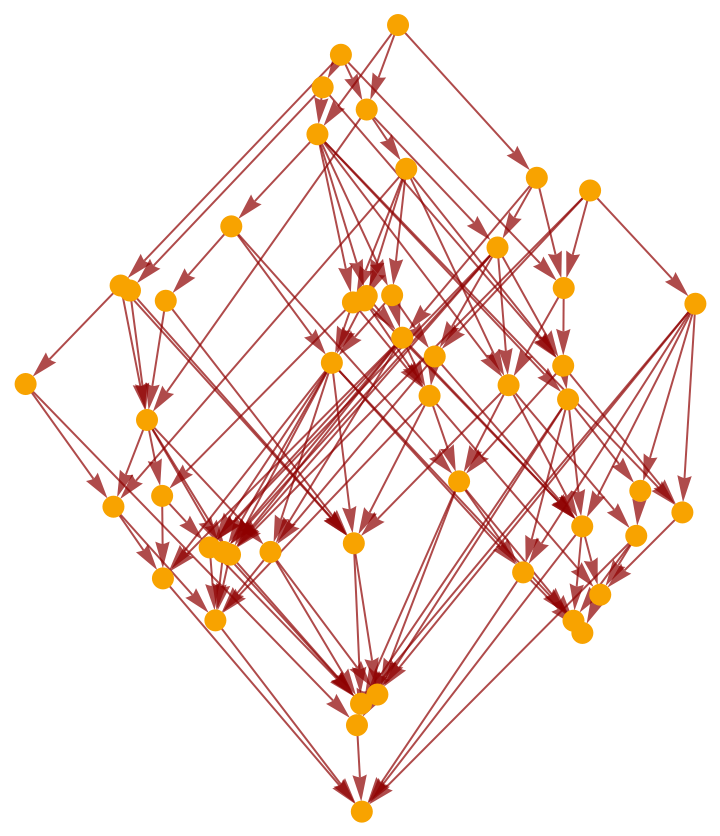Construct a subgraph of this causal graph corresponding to a smaller, interior diamond region:

 In:=Out=Compute the entanglement entropy associated with this subgraph using the naive approach:

 In:=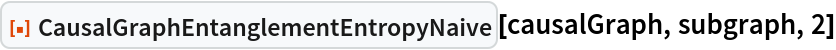Out=Highlight the interior subgraph within the larger (transitively-reduced) causal graph:

 In:=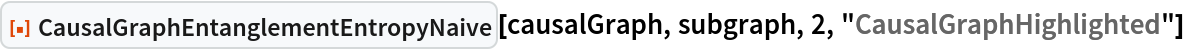Out=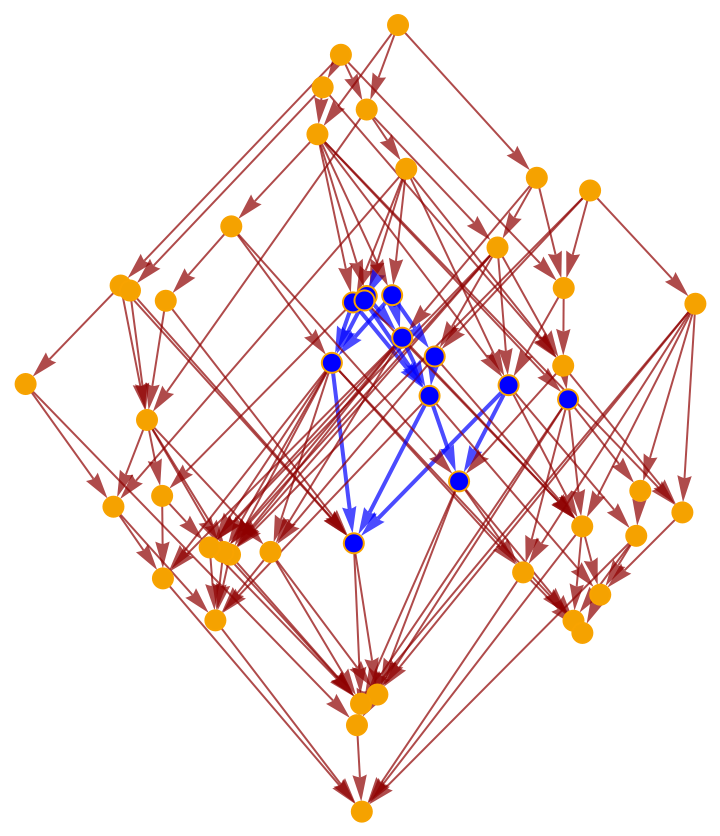Highlight the interior subgraph within the full (i.e. transitively-closed) causal graph:

 In:=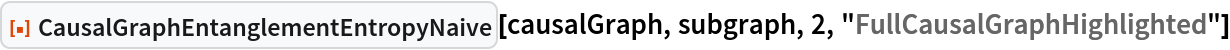Out=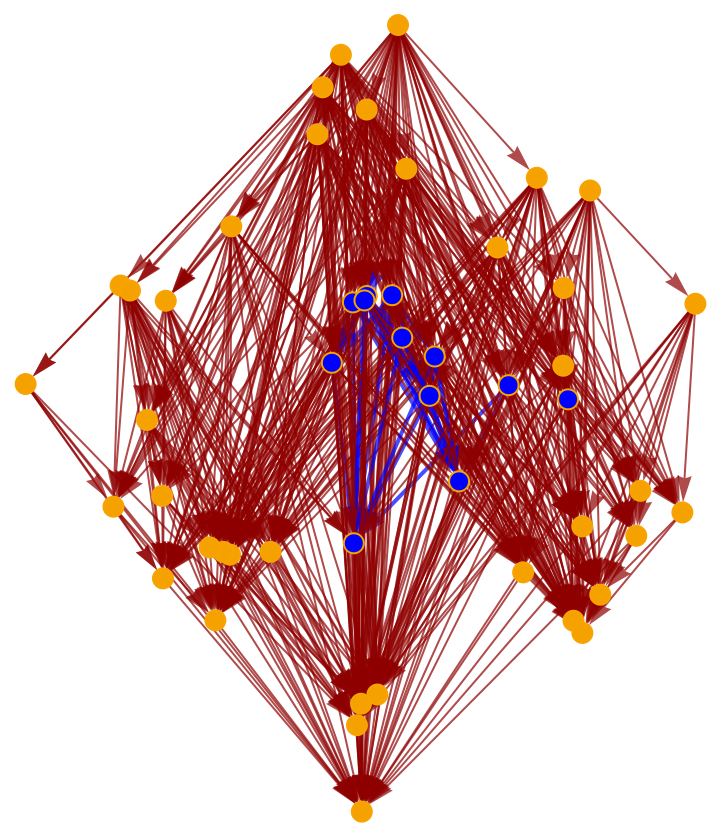Construct a causal graph by randomly sprinkling 100 points within a diamond-shaped region of 2+1-dimensional Minkowski space:

 In:=Out=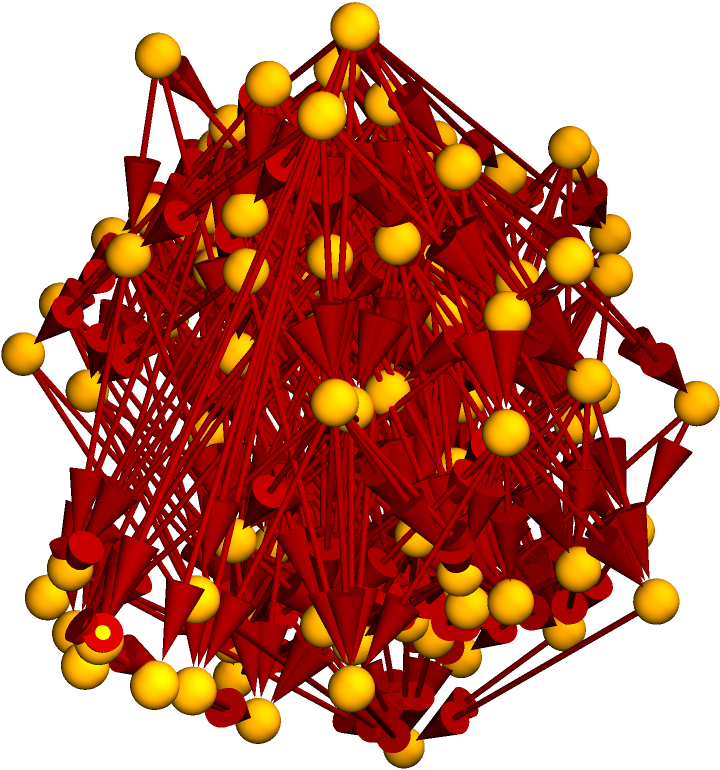Construct a subgraph of this causal graph corresponding to a smaller, interior diamond region:

 In:=Out=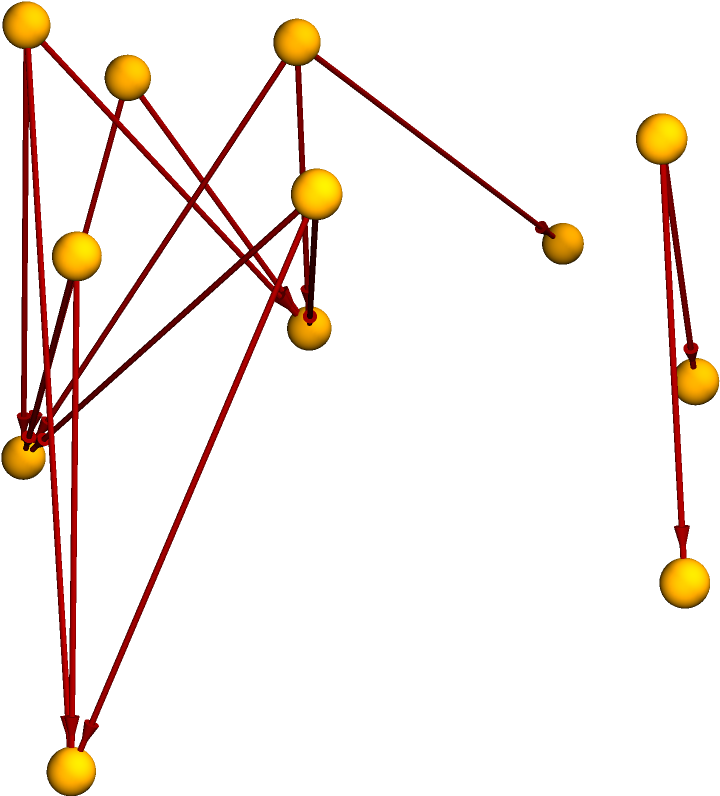Compute the entanglement entropy associated with this subgraph using the naive approach:

 In:=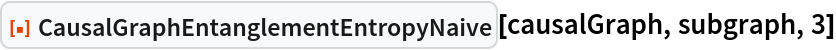Out=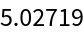Highlight the interior subgraph within the larger (transitively-reduced) causal graph:

 In:=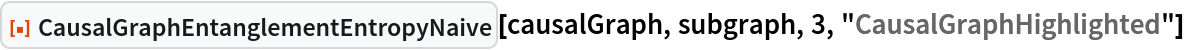Out=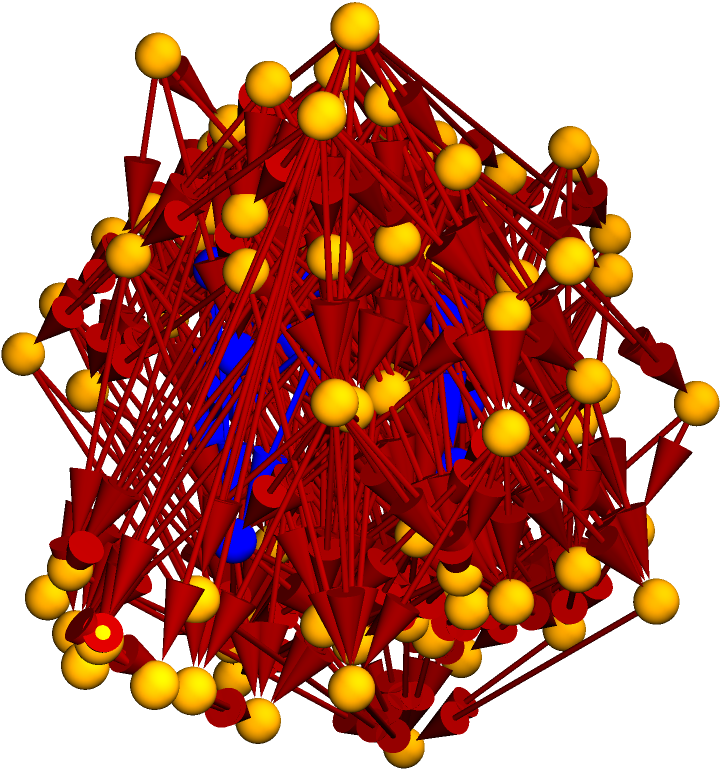Highlight the interior subgraph within the full (i.e. transitively-closed) causal graph:

 In:=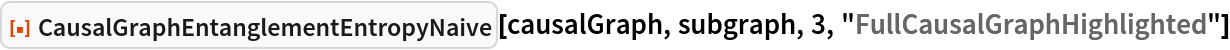Out=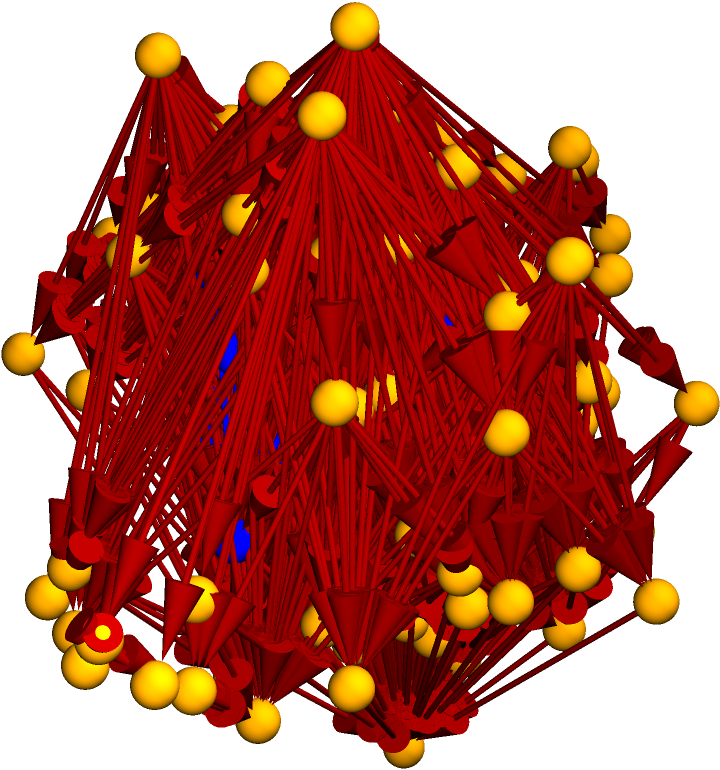Construct a causal graph by means of a deterministic algorithmic process, e.g. Wolfram model evolution:

 In:=Out=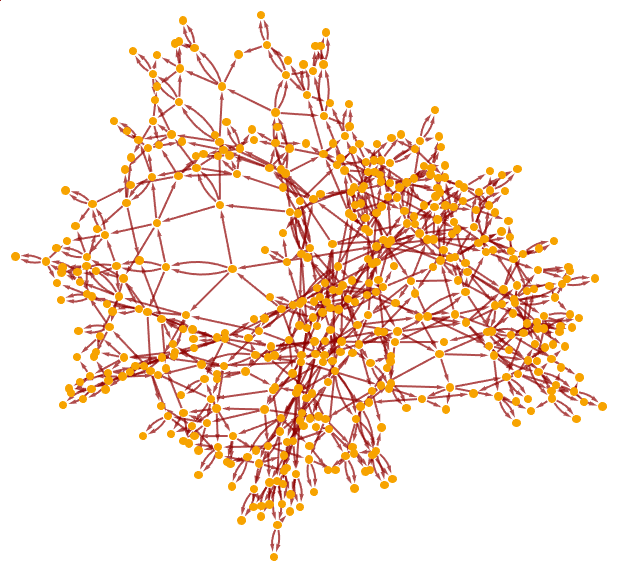Select a random subgraph of the resulting causal graph:

 In:=Out=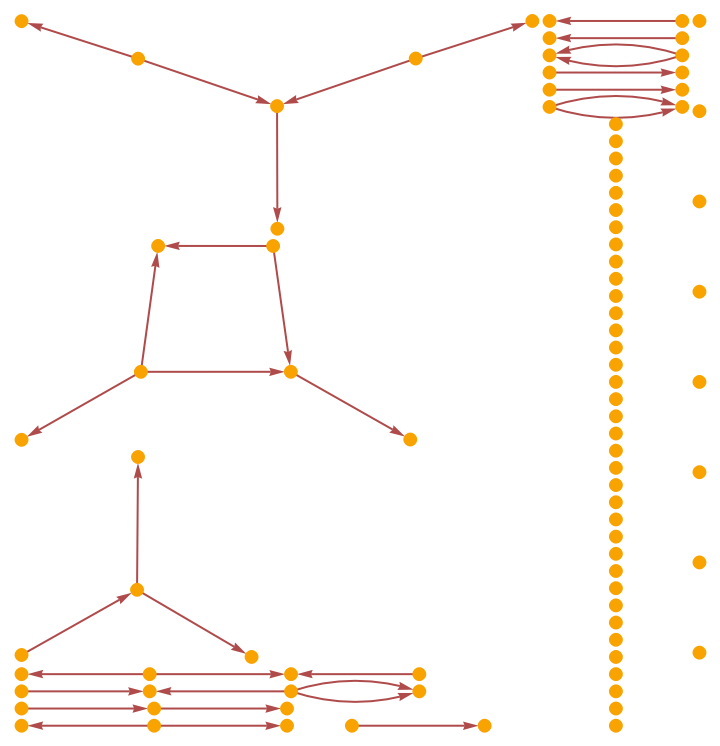Compute the entanglement entropy associated with this subgraph using the naive approach (note that, when an explicit dimension is not specified, CausalGraphEntanglementEntropyNaive will assume that the dimension of the causal graph is equal to 2):

 In:=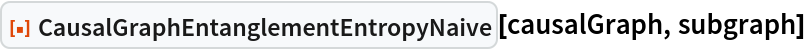Out=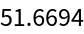Compute the entanglement entropy associated with this subgraph using the naive approach, assuming a causal graph dimension of 4 instead (yields an indeterminate result):

 In:=Out=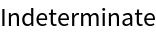Highlight the interior random subgraph within the larger (transitively-reduced) causal graph:

 In:=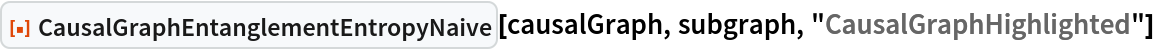Out=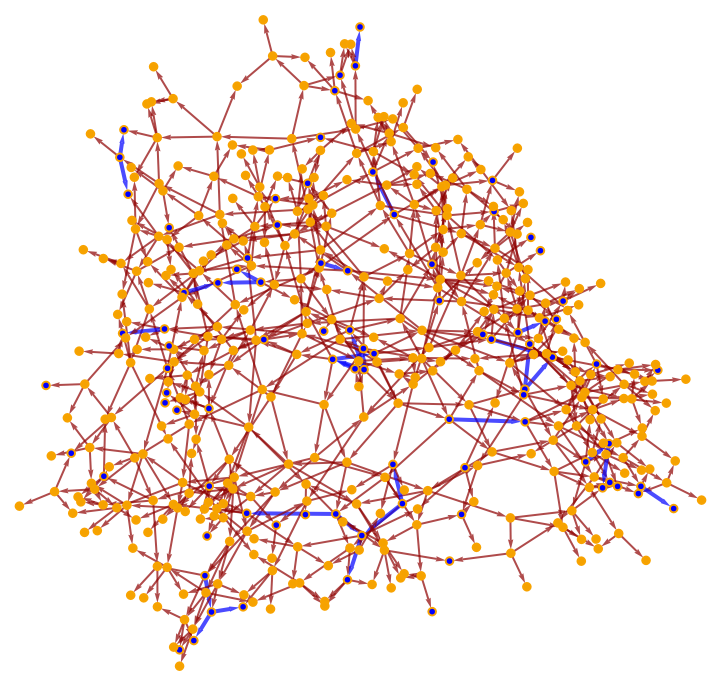When the Sorkin-Johnston Wightman function (or, equivalently, the Pauli-Jordan operator) of the corresponding causal set is not invertible, CausalGraphEntanglementEntropyNaive will return Indeterminate:

 In:=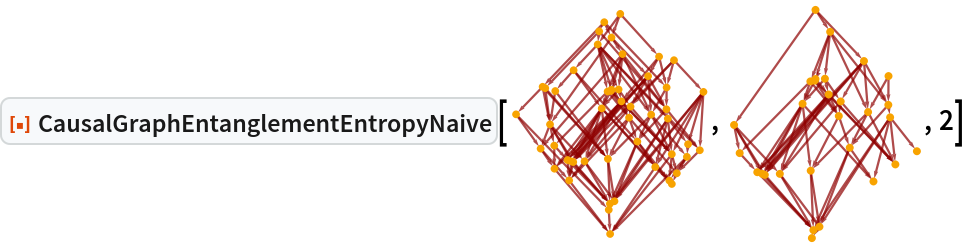Out=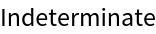Highlight the interior subgraph within the larger (transitively-reduced) causal graph:

 In:=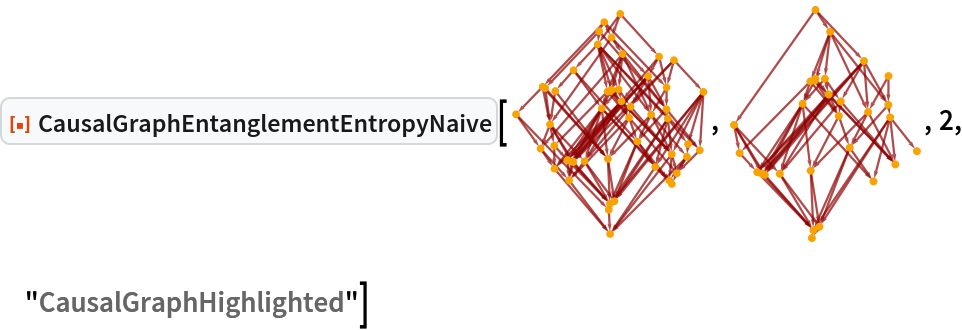Out=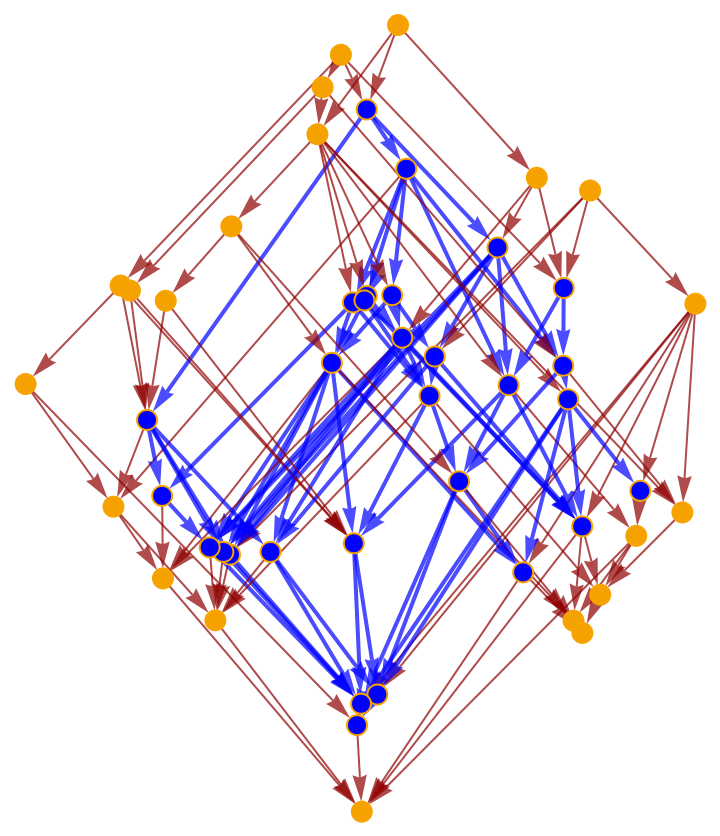### Scope (2)

CausalGraphEntanglementEntropyNaive currently supports the computation of causal set Green's functions (and hence entanglement entropies) in 1+1-dimensions:

 In:=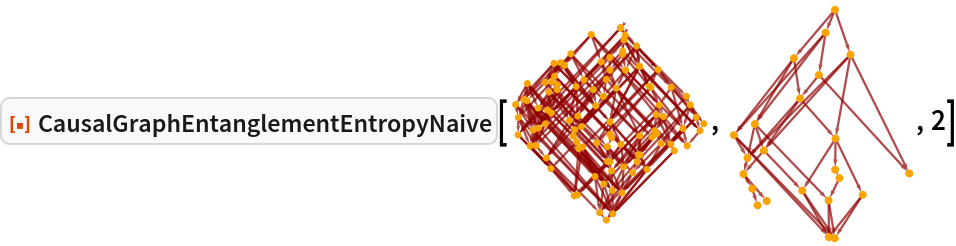Out=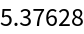2+1-dimensions:

 In:=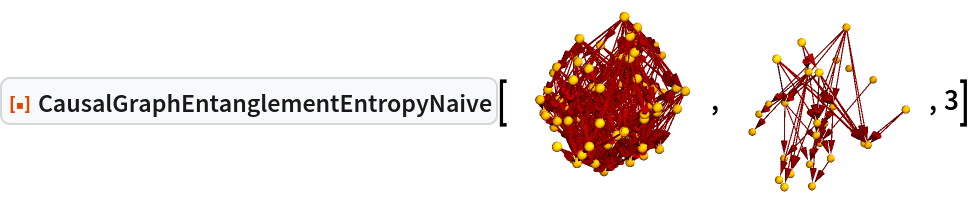Out=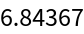And 3+1-dimensions:

 In:=Out=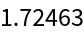When calling CausalGraphEntanglementEntropyNaive without any property specified, the property "EntanglementEntropy" is assumed by default:

 In:=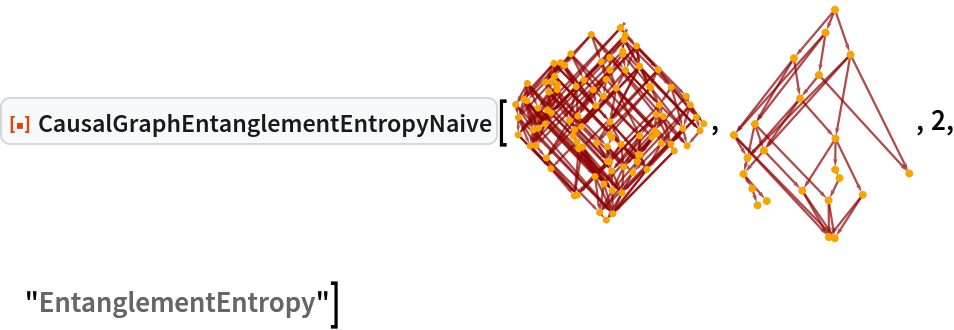Out=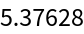Render the (transitively-reduced) causal graph:

 In:=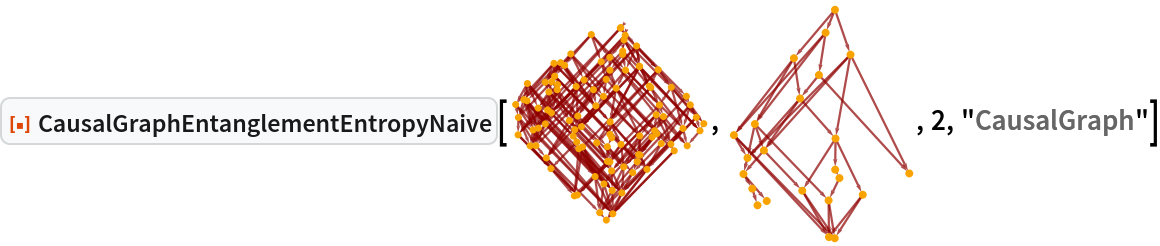Out=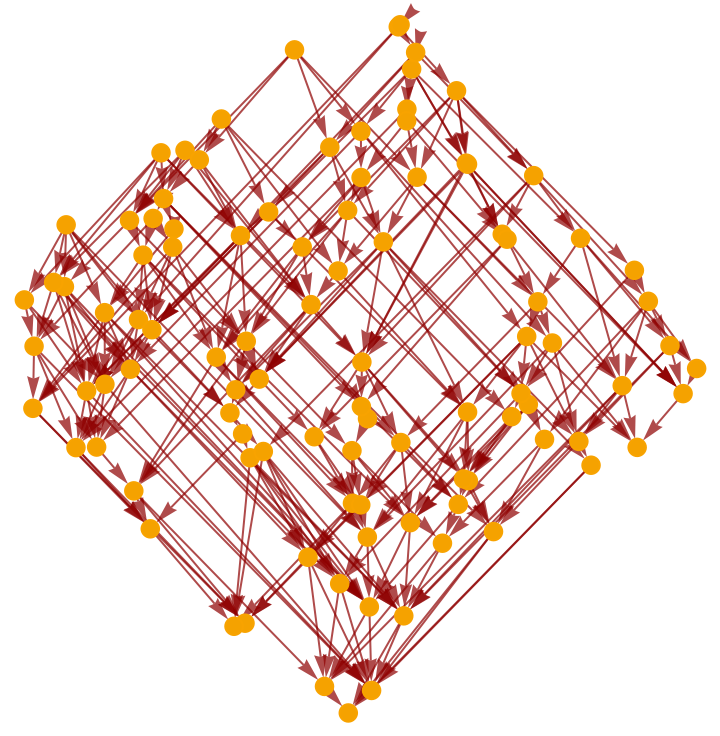Render the full (i.e. transitively-closed) causal graph instead:

 In:=Out=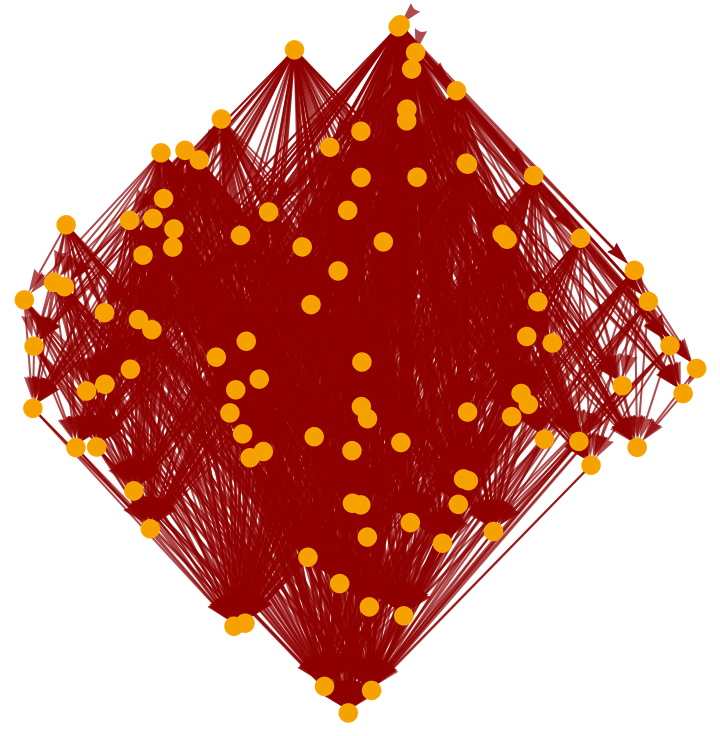Render the (transitively-reduced) interior subgraph of the overall causal graph:

 In:=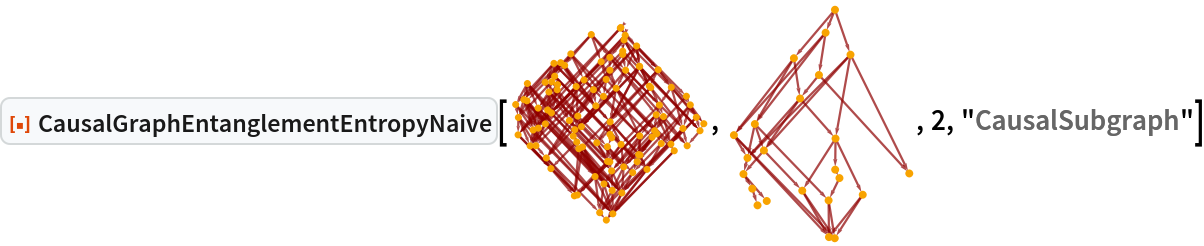Out=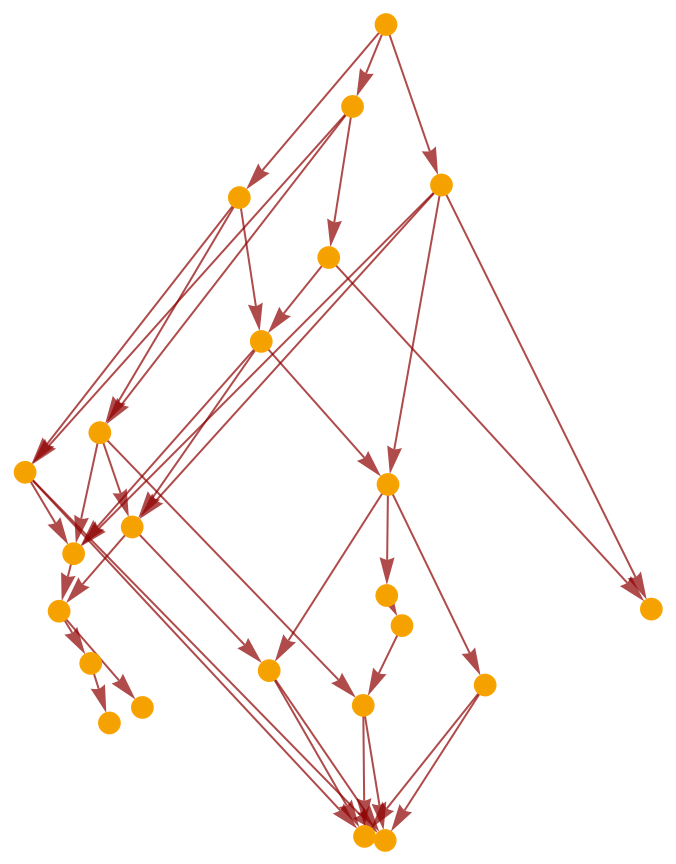Render the full (i.e. transitively-closed) interior subgraph of the overall causal graph instead:

 In:=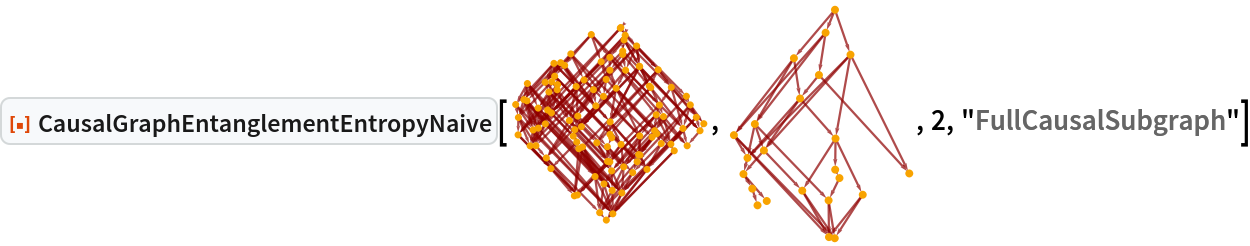Out=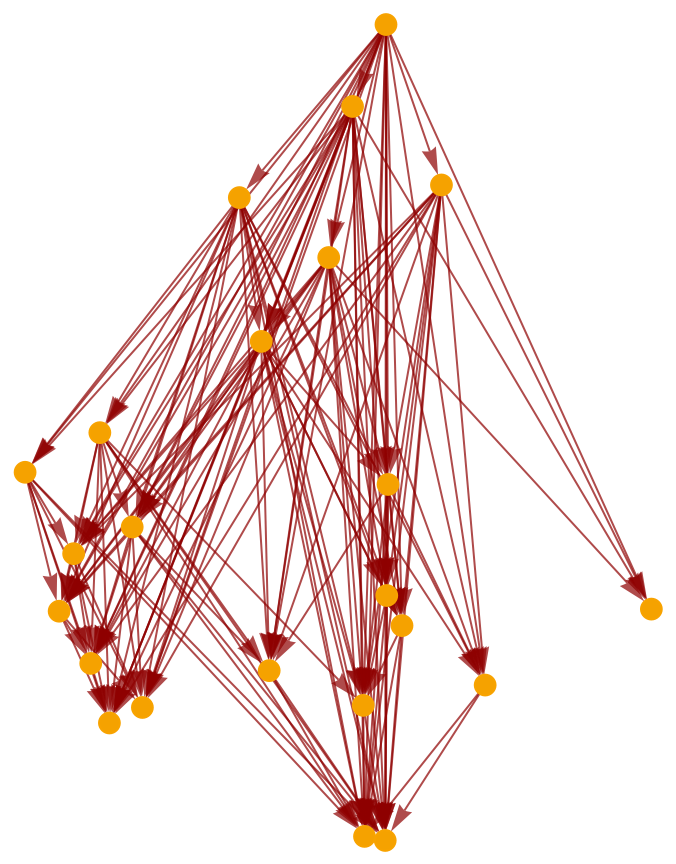Highlight the interior subgraph within the larger (transitively-reduced) causal graph:

 In:=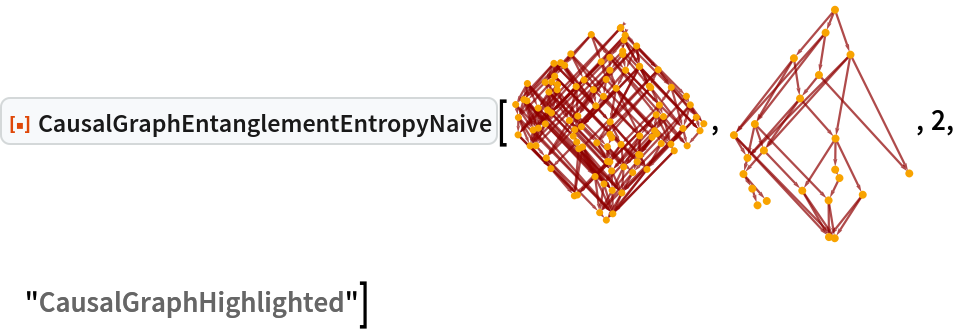Out=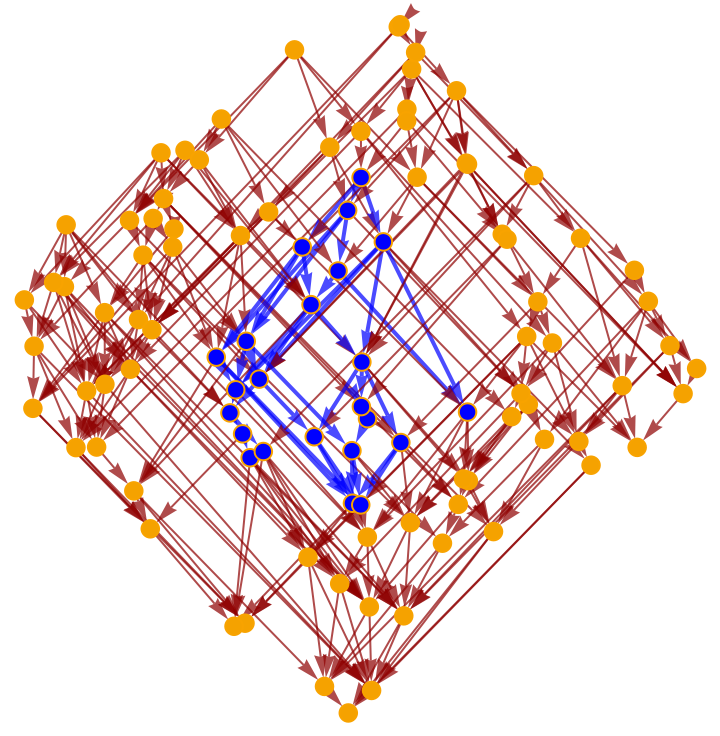Highlight the interior subgraph within the full (i.e. transitively-closed) causal graph instead:

 In:=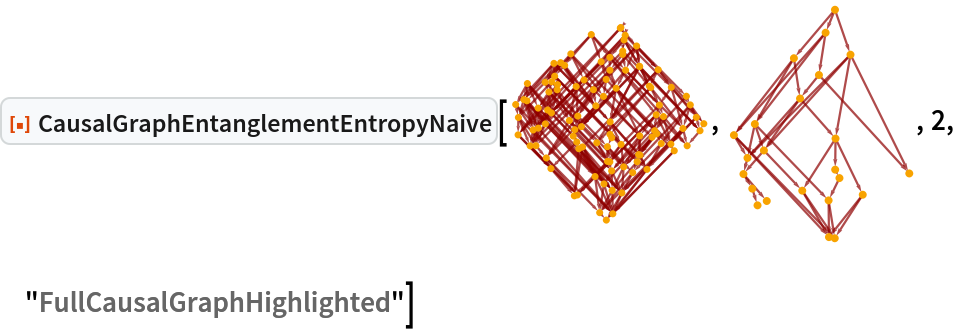Out=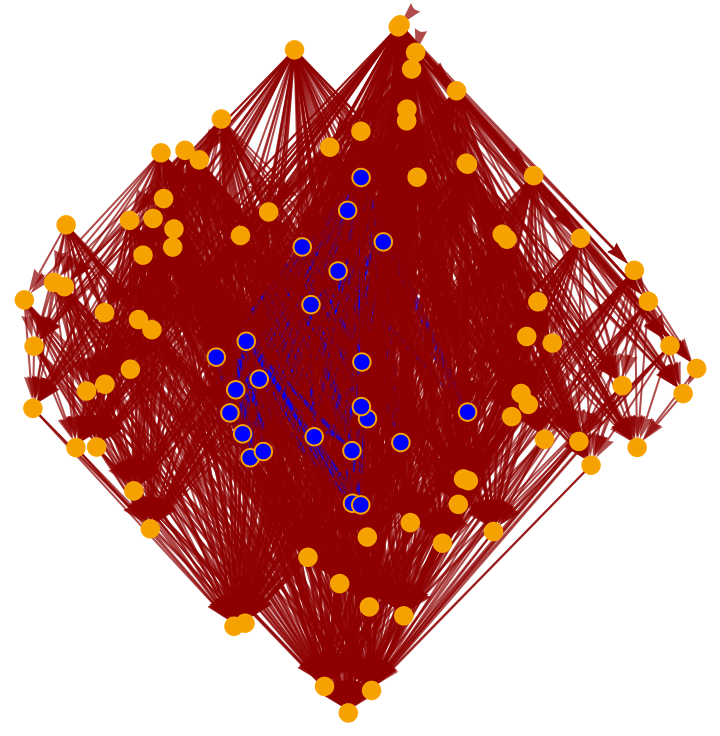Jonathan Gorard

## Version History

• 1.0.0 – 07 December 2021